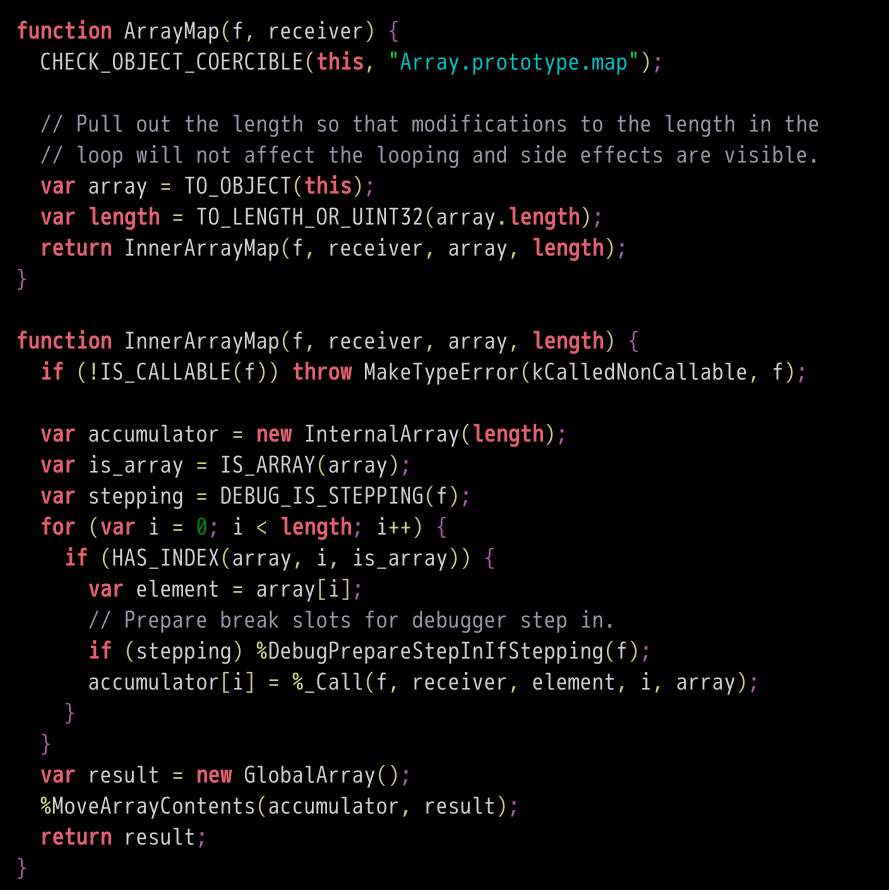# 读懂 ECMAScript 规格

## 一、概述ECMAScript 6的规格，可以在ECMA国际标准组织的官方网站（www.ecma-international.org/ecma-262/6.0/）免费下载和在线阅读。ECMAScript 6规格的26章之中，第1章到第3章是对文件本身的介绍，与语言关系不大。第4章是对这门语言总体设计的描述，有兴趣的读者可以读一下。第5章到第8章是语言宏观层面的描述。第5章是规格的名词解释和写法的介绍，第6章介绍数据类型，第7章介绍语言内部用到的抽象操作，第8章介绍代码如何运行。第9章到第26章介绍具体的语法。

## 二、相等运算符

``````
0 == null
``````

"The comparison `x == y`, where `x` and `y` are values, produces `true` or `false`."

1. ReturnIfAbrupt(x).
2. ReturnIfAbrupt(y).
3. If `Type(x)` is the same as `Type(y)`, then
Return the result of performing Strict Equality Comparison `x === y`.
4. If `x` is `null` and `y` is `undefined`, return `true`.
5. If `x` is `undefined` and `y` is `null`, return `true`.
6. If `Type(x)` is Number and `Type(y)` is String,
return the result of the comparison `x == ToNumber(y)`.
7. If `Type(x)` is String and `Type(y)` is Number,
return the result of the comparison `ToNumber(x) == y`.
8. If `Type(x)` is Boolean, return the result of the comparison `ToNumber(x) == y`.
9. If `Type(y)` is Boolean, return the result of the comparison `x == ToNumber(y)`.
10. If `Type(x)` is either String, Number, or Symbol and `Type(y)` is Object, then
return the result of the comparison `x == ToPrimitive(y)`.
11. If `Type(x)` is Object and `Type(y)` is either String, Number, or Symbol, then
return the result of the comparison `ToPrimitive(x) == y`.
12. Return `false`.

1. 如果`x`不是正常值（比如抛出一个错误），中断执行。
2. 如果`y`不是正常值，中断执行。
3. 如果`Type(x)``Type(y)`相同，执行严格相等运算`x === y`
4. 如果`x``null``y``undefined`，返回`true`
5. 如果`x``undefined``y``null`，返回`true`
6. 如果`Type(x)`是数值，`Type(y)`是字符串，返回`x == ToNumber(y)`的结果。
7. 如果`Type(x)`是字符串，`Type(y)`是数值，返回`ToNumber(x) == y`的结果。
8. 如果`Type(x)`是布尔值，返回`ToNumber(x) == y`的结果。
9. 如果`Type(y)`是布尔值，返回`x == ToNumber(y)`的结果。
10. 如果`Type(x)`是字符串或数值或`Symbol`值，`Type(y)`是对象，返回`x == ToPrimitive(y)`的结果。
11. 如果`Type(x)`是对象，`Type(y)`是字符串或数值或`Symbol`值，返回`ToPrimitive(x) == y`的结果。
12. 返回`false`

``````
0 == null // false
``````

## 三、数组的空位

``````
const a1 = [undefined, undefined, undefined];
const a2 = [, , ,];

a1.length // 3
a2.length // 3

a1 // undefined
a2 // undefined

a1 === a2 // true
``````

``````
0 in a1 // true
0 in a2 // false

a1.hasOwnProperty(0) // true
a2.hasOwnProperty(0) // false

Object.keys(a1) // ["0", "1", "2"]
Object.keys(a2) // []

a1.map(n => 1) // [1, 1, 1]
a2.map(n => 1) // [, , ,]
``````

"Array elements may be elided at the beginning, middle or end of the element list. Whenever a comma in the element list is not preceded by an AssignmentExpression (i.e., a comma at the beginning or after another comma), the missing array element contributes to the length of the Array and increases the index of subsequent elements. Elided array elements are not defined. If an element is elided at the end of an array, that element does not contribute to the length of the Array."

"数组成员可以省略。只要逗号前面没有任何表达式，数组的`length`属性就会加1，并且相应增加其后成员的位置索引。被省略的成员不会被定义。如果被省略的成员是数组最后一个成员，则不会导致数组`length`属性增加。"

## 四、数组的map方法

1. Let `O` be `ToObject(this value)`.
2. `ReturnIfAbrupt(O)`.
3. Let `len` be `ToLength(Get(O, "length"))`.
4. `ReturnIfAbrupt(len)`.
5. If `IsCallable(callbackfn)` is `false`, throw a TypeError exception.
6. If `thisArg` was supplied, let `T` be `thisArg`; else let `T` be `undefined`.
7. Let `A` be `ArraySpeciesCreate(O, len)`.
8. `ReturnIfAbrupt(A)`.
9. Let `k` be 0.
10. Repeat, while `k` < `len`
a. Let `Pk` be `ToString(k)`.
b. Let `kPresent` be `HasProperty(O, Pk)`.
c. `ReturnIfAbrupt(kPresent)`.
d. If `kPresent` is `true`, then
d-1. Let `kValue` be `Get(O, Pk)`.
d-2. `ReturnIfAbrupt(kValue)`.
d-3. Let `mappedValue` be `Call(callbackfn, T, «kValue, k, O»)`.
d-4. `ReturnIfAbrupt(mappedValue)`.
d-5. Let `status` be `CreateDataPropertyOrThrow (A, Pk, mappedValue)`.
d-6. `ReturnIfAbrupt(status)`.
e. Increase `k` by 1.
11. Return `A`.

1. 得到当前数组的`this`对象
2. 如果报错就返回
3. 求出当前数组的`length`属性
4. 如果报错就返回
5. 如果map方法的参数`callbackfn`不可执行，就报错
6. 如果map方法的参数之中，指定了`this`，就让`T`等于该参数，否则`T``undefined`
7. 生成一个新的数组`A`，跟当前数组的`length`属性保持一致
8. 如果报错就返回
9. 设定`k`等于0
10. 只要`k`小于当前数组的`length`属性，就重复下面步骤
a. 设定`Pk`等于`ToString(k)`，即将`K`转为字符串
b. 设定`kPresent`等于`HasProperty(O, Pk)`，即求当前数组有没有指定属性
c. 如果报错就返回
d. 如果`kPresent`等于`true`，则进行下面步骤
d-1. 设定`kValue`等于`Get(O, Pk)`，取出当前数组的指定属性
d-2. 如果报错就返回
d-3. 设定`mappedValue`等于`Call(callbackfn, T, «kValue, k, O»)`，即执行回调函数
d-4. 如果报错就返回
d-5. 设定`status`等于`CreateDataPropertyOrThrow (A, Pk, mappedValue)`，即将回调函数的值放入`A`数组的指定位置
d-6. 如果报错就返回
e. `k`增加1
11. 返回`A`

``````
const arr = [, , ,];
arr.map(n => {
console.log(n);
return 1;
}) // [, , ,]
``````

V8引擎对`map`方法的实现如下，可以看到跟规格的算法描述完全一致。[说明]：本文是我的新书《ECMAScript 6 入门》（第二版）的最后一章。

（完）

## 留言（17条）

8.函数的扩展函数的length属性

(function(a){}).length // 1
(function(a = 5){}).length // 0
(function(a, b, c = 5){}).length // 2

((x=0,y,z)=>x+y+z).length// 0

@Running_V：

coffeeScript 是根据那个标准来的

var a;
console.log(a);

var a;
a = 111;
console.log(a);

@Geek:

http://javascript.ruanyifeng.com/grammar/function.html#toc5

«-必填

«-必填，不公开

«-我信任你，不会填写广告链接